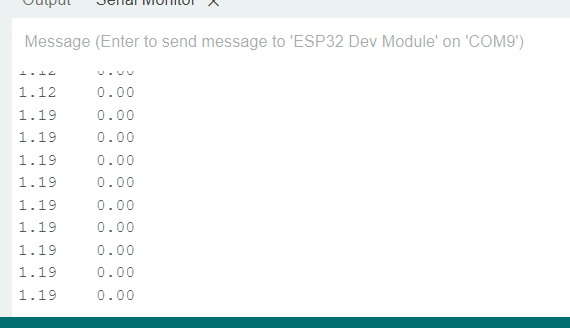# Hall sensor + esp32

Hello everyone, I am having problems controlling a BLDC motor. Specifically, when I checked the hallsensor, I found it was not working. Below is the code I use:
#include <SimpleFOC.h>
// #include <PciManager.h>
// #include <PciListenerImp.h>

HallSensor sensor = HallSensor(25, 26,13, 15);
// interrupt routine initialisation
void doA(){sensor.handleA();}
void doB(){sensor.handleB();}
void doC(){sensor.handleC();}
//PciListenerImp listenC(sensor.pinC, doC);

void setup() {
// monitoring port
Serial.begin(115200);
//
//sensor.pullup = Pullup::USE_EXTERN;

// initialise encoder hardware
sensor.init();
// hardware interrupt enabled
sensor.enableInterrupts(doA, doB, doC);
//PciManager.registerListener(&listenC);

_delay(1000);
}

void loop() {
// IMPORTANT
// read sensor and update the internal variables
sensor.update();
// display the angle and the angular velocity to the terminal
Serial.print(sensor.getAngle());
Serial.print(“\t”);
Serial.println(sensor.getVelocity());
delay(100);

}.
When I turn the wheel the angle is not rightWhat do you get if you replace the interrupt functions with:

``````void doA(){Serial.print("a");}
void doB(){Serial.print("b");}
void doC(){Serial.print("c");}
``````

If you don’t see anything printed then the interrupts aren’t interrupting.

It is a bit naughty printing to serial in an interrupt but it usually works for a character or two.

Also might be worth setting pinMode for your hall pins:

``````pinMode(25, INPUT);
pinMode(26, INPUT);
pinMode(13, INPUT);
``````

Do you actually have external pullups? A strong pull up resistor on each hall might be required for the halls to work, e.g. for hoverboard motors you might need a 1.5k resistor pulling up to 3.3v.

How do I set up the pull-up resistor?

Each of the three hall sensor output wires need a separate pullup. Connect them between the yellow, blue, green wires and 3.3V (red)

1 Like

Whilst it is possible to configure the esp32 hall pins as ‘internal’ pullups:

``````pinMode(25, INPUT_PULLUP);
pinMode(26, INPUT_PULLUP);
pinMode(13, INPUT_PULLUP);
``````

The internall pullup resistors are typically weak e.g. 30K ohms or more.

You might find that using input_pullup will work when the motor isn’t running (i.e. turning the motor by hand), but when you start the motor (which introduces noise) you get a lot of ghost interrupts - which gives glitchy velocity. So using an external pullup of 1 to 5K ohms will ensure a nice step transition as each hall passes through the magnetic fields of the permanent magnets of the rotor. If you have a 11pp motor then you’ll have 22 pole transitions which each hall can detect. So there should be 66 interrupts per rotation. If there is noise from the motor you can some times see 100s or 1000s of false interrupts happening. A strong pull up (e.g. a lower 1.5K resistor attached between 3.3v and each hall) will ensure that you get nice steps.

The motor I use has 15 pairs of poles, the pull-up resistor I use is 10k ohms. When the wheel rotates, it jerks. Is it because my PID parameters are not appropriate or due to some other reason?
motor.PID_velocity.P = 0.3f;
motor.PID_velocity.I = 0.3f;
motor.PID_velocity.limit = 0.04f;

Are you seeing stable/correct angles when you turn the motor? Are the angles correct i.e 1 rotation = 2pi?

Those pullups (at 10k) are still a bit weak, and may be prone to noise. For hoverboard motor halls i found 10k to glitch when motor was running and settled on 1.5k.

In terms of tuning, I’d start with zero for I and D and slowly increase P. You should initially see the motor speed lagging what you ask for and then when you find a value of P that gets close to your desired speed (70%) you might start to see speed wobbles. Only then would i play with D (to dampen wobbles) and i (to remove steady state error).

Note the d term is often a small value, much less than 1

Thank you bro, I will do that